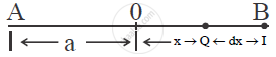# Obtan an Expression for Potential Energy of a Particle Performing S.H.M. What is the Value of Potential Energy at (I) Mean Position, and (Ii) Extreme Position - Physics

Sum

Obtan an expression for potential energy of a particle performing S.H.M. What is the value of potential energy at (i) Mean position, and (ii) Extreme position

#### Solution

Potential Energy :→ consider a particle of mass ‘m’ performing S.H.M along AB about mean position ‘O’.
→  OB = OA = a.
∴ When particle of mass ‘m’ performs S.H.M. is at distance x from its mean position.
→  Let ‘F = k x’ be the restoring force acting on the particle where k → force constant.
→  Let the particle is displaced by infinitesimal small distance 'dx'.
∴ Workdone,

dw =  - fdx= -(-kx) dx= kxdx.

∴  total workdone is ,

∫ dw= ∫ _(x=0)^x kxdx =k  ∫ _(x=0)^x xdx

W = 1/2 kx^2= 1/2 mω^2x^2            [∵ ω^2 = k/m]

∴ this workdone is stored in the form of P.E.

∴ P.E = 1/2 kx^2 = 1/2mω^2x^2

Cases :
(i) at Mean position : - x = 0

∴ P.E = 0

(ii) at extreme position : x = ± a

∴ magnitude of P.E. is,

∴ P.E =1/2 mω^2a^2

Concept: K.E.(Kinetic Energy) and P.E.(Potential Energy) in S.H.M.
Is there an error in this question or solution?